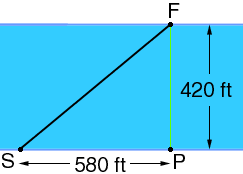My math question is: Bob swam across a river 420 ft wide. A strong current carried him 580ft downstream as he swam. Find x, the distance bob actually swam. Hi Torri, Draw a diagram!Bob started at S anf finished at F, which is 580 ft downstream. The triangle PFS is a right triangle so you can use the theorem of Pythagoras to find the hypotenuse, SF. Penny Go to Math Central